## Numerical Optimal Control in Continuous Time Made Easy

Eric Naevdal (*)
Research Fellow
Department of Forest Sciences
Agricultural University of Norway

### Abstract

The paper explains how continuous time optimal control models can be solved with Microsoft Excel. Two models are solved, each illustrating a different aspect of the Optimal Control Theory. The first is a model of real investment. The second is a model of fisheries management. The investment model is used to illustrate how one can simultaneously maximise the Hamiltonian and solve the differential equations for the state and the co-state variables, a computational aspect overlooked in the literature. The paper is aimed at researchers looking for a way of doing numerical optimal control in continuous time without being proficient in programming or advanced mathematical software.

JEL codes: C61, C63
Keywords: optimal control theory, numerical methods, trapezoid method

### Introduction

Optimal control (OC) is a basic tool in the economist's toolkit. Standard models in consumer theory, investment theory and resource economics are often developed within an OC framework. It well known that in general OC models have no closed form solutions. If one desires more than qualitative results, numerical methods are required to solve these models. It is therefore strange that in the economic literature, disregarding numerical analysis on closed form solutions, numerical solutions to OC problems are few. In recent years, papers by Tahvonen and Withagen (1996), Tarkainen and Tuomala (1999), Ehlgen (1999), Kunkel and von dem Hagen (2000) and Salo and Tahvonen (2001) are among the exceptions. Most of these papers deals with algorithms rather than applications.

In most graduate level courses covering OC and economic dynamics, students receive training in comprehensive training in the analytic and qualitative OC, but not in the numerical methods required to solve real world problems. The present paper tries to modify this state of affairs by introducing a method that allows a student to solve OC problems using the Solver Tool in Microsoft Excel in a few easy steps. The Solver Tool, bundled with Microsoft Excel and developed by Frontline Systems, is one of the most versatile solvers available for solving non-linear equations and numerical optimisation problems.(note1) Some applications that utilise this tool exist in the literature, e.g. Førsund and Nævdal (1998), as well as in recent textbooks where simple dynamic programming models are solved in order to illustrate the theory, e.g. Conrad (1999).

The paper is organised as follows. First, there is a section on how to set up an OC problem in Excel by using a method called the Trapezoid method. The second section analyses two OC models, each illustrating a different aspect of the optimal control theory. The first is a model of real investment, where optimal investment schedules are derived in the simplest possible model. The model is solved both numerically and analytically for a quick comparison of the two techniques. The second is a model of fisheries management. This model is highly non-linear and cannot be solved analytically. The third section shows how one can solve problems where one cannot analytically maximise the Hamiltonian. The investment models is then revisited in order to illustrate how one can simultaneously maximise the Hamiltonian and solve the differential equations for the state and the co-state variables. This is a computational aspect of numerical OC overlooked in the literature.

In the rest of this article, it is assumed that readers are familiar with analytical OC theory. Readers looking for an introduction to the topic are directed to Seierstad and Sydsæter (1987). For instructors who are interested in applying the methods below, the models analyzed in this article are available from the author on request.

### Optimal Control Theory and Numerical Methods

OC problems come in a number of different varieties. The most basic with scalar control and state variable, is:

(1)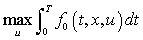(2)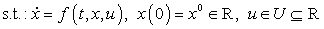Pontryagin's Maximum Principle, (see Seierstad and Sydsæter (1989) for details) gives necessary conditions for this problem. These conditions are usually presented in terms of the Hamiltonian defined by:

(3)      H(t,x,u,p) = f0(t,x,u) + pf(t,x,u)

The necessary conditions are:

(4)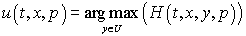(5)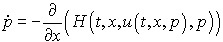(6)(7)      p(T)=0

The problem defined by equations (5) to (7) and the condition x(0) = x0 is an instant of a more general class of problems, called Boundary Value Problems (BVPs). The general form (when z is a vector in Rn) is

(8)(9)      h(z(0), z(T))=0

There is a large mathematical literature concerned with solution techniques for BVPs. A good introduction is found in Ascher and Petzold (1998). For a discussion aimed at economists, see Judd (1998). Two types of algorithms are widely used. One method is called "shooting". This technique is troubled by issues of instability caused by the saddle point property of steady states in many OC problems. Also, the method is computationally expensive if the Hamiltonian must be maximised numerically. Here we use a finite difference method called the Trapezoid method. The method is well known in the mathematical literature, although, to my knowledge, the method has not been applied in the economic literature. Here we note that if we define a mesh (0, h, 2h, ×××, nh) on the interval [0,T], with h = T/n we can define a set of equations that provide a set of values z(tn) approximating the true values of z if such values exist. The system of equations is given by (9) and:

(10)Writing out (10) in the case where the system is an OC problem gives us:

(11)(12)After inserting un = argmaxu H(tn, xn, u, pn) and un-1 = argmaxu H(tn-1, xn-1, u, pn-1), equations (11) and (12) provides us with 2(n-1) equations for the 2n unknowns {x}n and {p}n. x(0) = x0 and p(T) = 0 provides us with two additional equations required to determine the system. A complication occurs when (4) does not yield an explicit solution for u(t, x, p). Then we must add n more equations so that we simultaneously solve (11), (12), x(0) = x0, p(T) = 0 and the following n equations.

(13)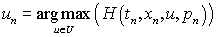The trick is to find a way of implementing the algorithms here described. The rest of the paper illustrates by example how this can be done very quickly in Microsoft Excel.

### Optimal Control Models

#### Real Investment

Consider the following model. A firm has a production y = xa. Here y is output and x is capital. The stock of capital is assumed to be driven by the differential equation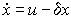. Here u is investment and d is the rate of capital depreciation. Assume that the cost of I is given byu2 and the market price of y is equal to one. This leads to the following maximisation problem.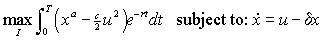Solving this model with c = a = d = T = 1, r = 0 and assuming x(0) = 0, gives us the following necessary conditions

(14)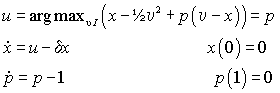The analytical solution to this problem is given by u = p = 1-et-1 and x = et - ½e2t-1 + (½e-1 - 1)e-t. We now proceed to solve this model numerically. First, the differential equations in (14) are programmed as functions in Excel's macro language. How this is done is illustrated in the appendix. The defined functions should look something like this:

```Function f(t, x, p)
f = p - x
End Function

Function dp(t, x, p)
dp = -1 + p
End Function
```

Then we enter the model into the spreadsheet. One way of doing this is shown in Figure 1. Note that the formulas are displayed by a special option that allows the display of formulas in the spreadsheet rather than the numbers usually shown. The step size, h, is entered into the cell B1. In cell C2, time is zero. Time goes horizontally, so in cell D2 time is equal to 0 + step size. In cell C3 and D3 are initial guesses at the state variable and for time t = 0 and time t = 0 + step size. Finally in D5 the formula from (11) is entered for n = 1. In D6, (12) is entered for n = 1. The next step is to copy the contents of cells D2-D6 to E2-E6, F2-F6 and so on until the cell W2 show the value 1. How the formulae are entered into the Spreadsheet is illustrated in Figure 1.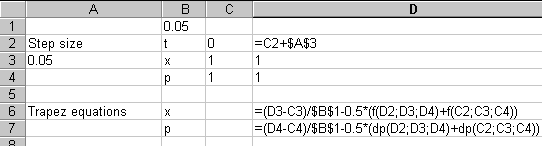Figure 1: Entering Formulae for the Investment Problem

This concludes the necessary "coding" in order to solve the problem. It remains to set up the problem in Excel's Solver application. The solver is asked to find values in the cells C3-W3 and C4-W4 that makes p(1) in cell W4 equal to 0. The solution is constrained by the initial stock of capital, x(0) in cell C3 should be equal to zero since x(0) = 0 by assumption. The equations in cells D5-W5 and D6-W6 represents the Trapezoid equations from equations (11) and (12), and should be equal should be equal to zero. If we examine the problem closely, we see that in this model, equations (11) and (12) are in fact linear with respect to the variables xn, xn-1, pn and pn-1. The solution process may therefore be made faster and more reliable by checking the 'Assume Linear Model' check box in the Solver Options dialog box. After setting the up the problem, the Solver dialog box should look like Figure 2.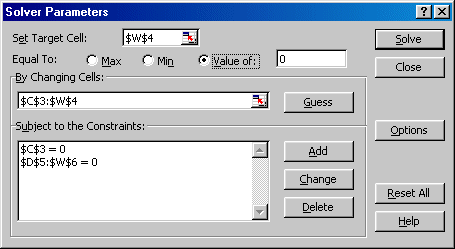Figure 2: Solver Dialog Box for Investment Problem

Pressing Solve solves the problem. The resulting capital stocks and co-state variables are displayed in Table 1.

Table 1: Real Investment Example, Solution

t Numerical Estimates Exact Solutions Trapezoid equations
Capital Co-State Capital Co-State xp
0 0 0.63219722 0 0.632120559
0.05 0.03037885 0.61333554 0.03036894 0.613258977 0 -1.38778E-15
0.1 0.05833213 0.59350659 0.05831329 0.59343034 0 1.77636E-15
0.15 0.08392976 0.57266078 0.08390294 0.572585068 0 -7.21645E-16
0.2 0.10723579 0.55074595 0.10720187 0.550671036 0 0
0.25 0.12830852 0.52770728 0.12826834 0.527633447 00
0.3 0.14720065 0.50348714 0.14715502 0.503414696 0 7.21645E-16
0.35 0.16395945 0.47802494 0.16390915 0.477954223 0 0
0.4 0.17862684 0.45125699 0.17857262 0.451188364 0 0
0.45 0.19123951 0.42311632 0.19118209 0.42305019 0 -8.88178E-16
0.5 0.20182902 0.39353254 0.20176909 0.39346934 0 0
0.55 0.21042185 0.36243165 0.2103601 0.362371848 2.77556E-16 0
0.6 0.21703951 0.32973583 0.2169766 0.329679954 0 0
0.65 0.22169853 0.29536331 0.22163513 0.29531191 -2.91434E-16 0
0.7 0.22441059 0.2592281 0.22434735 0.259181779 -6.93889E-17 0
0.75 0.22518246 0.22123979 0.22512003 0.221199217 -2.77556E-17 0
0.8 0.22401608 0.18130337 0.2239551 0.181269247 6.93889E-17 0
0.85 0.22090852 0.13931893 0.22084966 0.139292024 -5.55112E-17 0
0.9 0.21585202 0.09518144 0.21579594 0.095162582 3.33067E-16 0
0.95 0.20883392 0.04878049 0.2087813 0.048770575 0 0
1 0.19983666 0 0.1997882 0 0 0

As expected, the capital stock, x, first increases in order to increase production. Capital is accumulated until t = 0.75. Thereafter the short remaining time horizon makes it optimal to let capital depreciate at a higher rate than the rate of gross investment, u. This follows from the co-state variable being a continuously decreasing function of time and the transversality condition forcing p(1) = 0. In the table, the exact solution is also given for both variables. The largest discrepancy between the numerically estimated capital stock and the estimated one is 0.000063. For the co-state the largest difference is 0.000077. The method thus appears to be reasonably accurate and the errors are well below what would be considered satisfactory in most economic applications. The left hand side of equations (11) and (12) is shown in the table. It is evident that for both the state and the co-state (11) and (12) hold almost exactly for all values of t.

#### Fisheries Management

A standard model in any resource economics class is optimal fisheries management. The classical model is built on the Schaefer harvesting model that assumes that the growth rate of a stock of fish is a quadratic function of the stock and a linear function of harvesting effort. Clark (1976) provides a thorough discussion. Unfortunately applying OC to the Schaefer model yields no closed form solutions. Using the previous model as a template, it takes minutes to find numerical estimates. A few more issues will be illustrated. First it is assumed that there are restrictions on how small the stock of fish is allowed to be at the end of the planning horizon in order to illustrate how endpoint constraints may be incorporated. Second, we show how non-negativity constraints on the control may be incorporated. Third, it is shown how to simplify the functional definitions in order to simplify changes in the model. Let h be the harvesting rate, x be the stock of fish, r the interest rate, p is the price of fish, K is the carrying capacity, c is the marginal cost of effort in a quadratic cost function and q is the catchability coefficient. The control variable is harvesting effort, E. Effort is assumed to be related to harvesting by h = qxE. The problem to be solved is:

(15)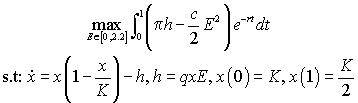It is assumed that the fishery starts out in the steady state without harvesting and that quotas are set so that at the end of the planning horizon the stock of fish should be equal to half of the carrying capacity. In order to solve this problem we first find the necessary conditions by using the maximum principle. It is assumed that we are analysing a previously unexploited resource so x(0) = K. The Hamiltonian to this problem is given by: H(t, x, p, E) = (pqxE - (c/2)E2)e-rt + p(x(1 - x/K) - qxE). Again p is the co-state variable. The optimal extraction, E(t, x, p) equals argmaxE (H(t, x, p, E)) =(p-pert) unless the requirement that E Î [0,2.2] is binding in which case E is given by the binding boundary. The differential equations along the optimal path are given by:

(16)(17)If a copy of the previous problem is used, time is saved because the trapezoid equations are already entered into the spreadsheet. Coding the differential equations into Excel's macro language may be done as follows:

```Public Const q = 1
Public Const Pi = 1
Public Const r = 0.4
Public Const c = 2
Public Const K = 20

Function f(t, x, p)
E = ((q * x) / c) * (Pi - p * Exp(r * t))
If E < 0 Then E = 0
ElseIf E > 2.2 Then E = 2.2
End If
f = x * (1 - (x / K)) - q * x * E
End Function

Function dp(t, x, p)
E = ((q * x) / c) * (Pi - p * Exp(r * t))
If E < 0 Then E = 0
ElseIf E > 2.2 Then E = 2.2
End If
dp = -Pi * q * E * Exp(-r * t) - p * (1 - (2 * x / K)) + p * q * E
End Function
```

Note how the written code is almost identical to how (16) and (17) are written. The first section of code defines the constants used in the solution. It makes sense to define them this way rather than entering numbers directly in the function because it simplifies entering the formulas and vastly simplifies the process of finding solutions with different parameter values. Instead of retyping the code, just change the number where the constant is defined. The next two sections define the differential equations. Note how the boundary of the control region is accounted for. (note2) The way the boundary is accounted here is rather ad hoc, but works with the present problem. If a copy of the spreadsheet from the previous example was used, the problem may then be solved. After loading the Solver application only a minor modification must be made. Instead of setting p(1) to zero, we here set x(1) to K/2, which in this particular case is equal to 10. This is done by changing the Set target cell to \$W\$3 and require that this cell should take the value 10. The Solver Dialog Box should look like Figure 2.Figure 3: Solver Dialog Box for Fisheries Example

Again, pressing Solve starts the calculations. The solution found by Excel is given in table 2. Note that we also reproduce the optimal values of E. Once the optimal values of x and p are found it is easy to calculate E(t, x, p), and the values of E are also reported.

Table 2: Fisheries - Numerical Estimates

t x p E
0 20 0.711729 2.2
0.05 17.95814 0.71317 2.2
0.1 16.23452 0.710516 2.114439
0.15 14.85741 0.70597 1.859965
0.2 13.79658 0.701017 1.659713
0.25 12.96006 0.695779 1.497183
0.3 12.2891 0.690362 1.361752
0.35 11.74447 0.684859 1.24623
0.4 11.29906 0.679356 1.145547
0.45 10.93363 0.673927 1.055983
0.5 10.63426 0.668644 0.974717
0.55 10.39076 0.66357 0.89953
0.6 10.19565 0.658768 0.828612
0.65 10.04346 0.654292 0.760432
0.7 9.930311 0.6502 0.693639
0.75 9.853624 0.646541 0.62699
0.8 9.811908 0.643367 0.559288
0.85 9.804681 0.640725 0.489329
0.9 9.832435 0.638662 0.41585
0.95 9.896669 0.637222 0.337473
1 10 0.63645 0.252637

Note how control region is binding for the two first time periods. In this example the solution is likely to be less accurate than in the Investment example since the differential equations are non linear. In non-linear models, a smaller step-size is usually appropriate.

One get a pretty good indication of how an optimal solution will look by using Excel's graphing capabilities. This is done in Figure 4. Note in Figure 4 how the stock of fish dips below the required end value x(1) = 10. The interpretation of this is that when facing an intertemporal trade-off between preserving today and in the future it is better to harvest today and let the stocks replenish themselves tomorrow.Figure 4: Stocks and Co-State Along the Optimal Path

### Simultaneously Solving For the Control Variables

So far we have found the control maximising the Hamiltonian analytically. This is often the case. However, occasionally this is not possible and the maximisation of the Hamiltonian must be done numerically. This is a complication that is straightforward to handle with Excel. Assuming that the control may be chosen freely in the necessary condition that must be incorporated into Excel is simply.

(18)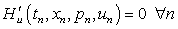Let us solve the real investment problem from section one so that we may compare with the solution found there. The necessary conditions are:

(19)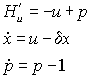We must know rewrite the functions in Excel since u is not eliminated analytically. We must also define a function that evaluates the derivative of the Hamiltonian with respect to u. The functions now look like this.

```Function f(t, x, p, u)
f = u - x
End Function

Function dp(t, x, p, u)
dp = -1 + p
End Function

Function dHdu(t, x, p, u)
dHdu = -u + p
End Function
```

We must then rewrite the formulae in the spreadsheet in order to account for the changes in the function definitions. This is done in Figure 5. The cells in row 4 now show the value of the control. Here they are arbitrarily set to 1 since the optimisation has not been performed yet. References to the f function and the dp functions now take four arguments. The last argument refers to a cell with a control variable. Finally, row 8 calls the dHdu function. After making these modifications we are ready to set up the Solver.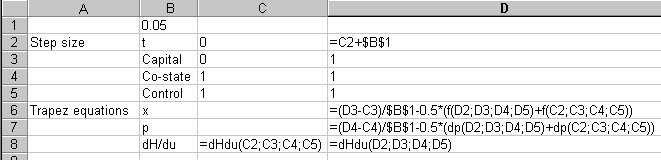Figure 5: Cells for the Real Investment Problem with numerically estimated controls.

After making these modifications, we are ready to load the Solver and find the solution. The Solver dialog box should look like Figure 6.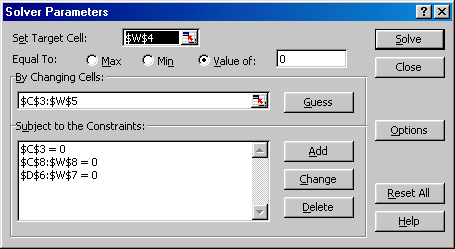Figure 6: Solver Dialog for Investment Problem with Estimated Control

Table 3: Solution for Investment problems with Estimated Control

t Capital Co-state Control Capital Co-state Solution with Numerically Estimated u Solution with Analytically Determined u 0 0 0.63219722 0.63219722 0 0.63219722 0.05 0.030378848 0.61333554 0.61333554 0.03037885 0.61333554 0.1 0.058332127 0.59350659 0.59350659 0.05833213 0.59350659 0.15 0.083929764 0.57266078 0.57266078 0.08392976 0.57266078 0.2 0.107235793 0.55074595 0.55074595 0.10723579 0.55074595 0.25 0.128308516 0.52770728 0.52770728 0.12830852 0.52770728 0.3 0.147200647 0.50348714 0.50348714 0.14720065 0.50348714 0.35 0.163959447 0.47802494 0.47802494 0.16395945 0.47802494 0.4 0.178626838 0.45125699 0.45125699 0.17862684 0.45125699 0.45 0.191239512 0.42311632 0.42311632 0.19123951 0.42311632 0.5 0.20182902 0.39353254 0.39353254 0.20182902 0.39353254 0.55 0.210421853 0.36243165 0.36243165 0.21042185 0.36243165 0.6 0.217039506 0.32973583 0.32973583 0.21703951 0.32973583 0.65 0.221698533 0.29536331 0.29536331 0.22169853 0.29536331 0.7 0.22441059 0.2592281 0.2592281 0.22441059 0.2592281 0.75 0.225182461 0.22123979 0.22123979 0.22518246 0.22123979 0.8 0.224016077 0.18130337 0.18130337 0.22401608 0.18130337 0.85 0.22090852 0.13931893 0.13931893 0.22090852 0.13931893 0.9 0.215852016 0.09518144 0.09518144 0.21585202 0.09518144 0.95 0.208833915 0.04878049 0.04878049 0.20883392 0.04878049 1 0.199836663 0 0 0.19983666 0

Note that the only difference from the Solver Dialog Box in Figure 1 is that we have added the constraint that the derivatives of the Hamiltonians in cells \$C\$8:\$W\$8 should equal zero. Again, pressing Solve gives us the solution. The estimated values are given in Table 3. If we compare the two solutions, we see that p and u are identical in the two models. For the x variable, there are small discrepancies, but the differences are minute.

This model was nice in the sense that the differential equations and the derivative of the Hamiltonian are all linear. With less linear models, the results will be less exact.

### Tips, Tricks and Limitations

This section contains a few notes that may be helpful when implementing the method here described.

#### Solver Limitations

The most serious limitation when solving OC problems the way described here lies in the limitations of the Solver supplied with the Microsoft Excel. The Solver only allows for 100 unknowns, which is a severe limitation when solving larger OC problems. If we solve a problem where we have an analytical solution for the control as a function of state and co-state variables and only one state variable, we can only solve problems over the interval [0, 49×step size]. There are two ways around this problem. One is to use a shooting algorithm rather than the trapezoid method. When this algorithm works, it works well. Implemented in Excel it is in fact faster than the trapezoid method. But the Shooting algorithm will often not work very well due to numerical instability. The second way is to use an extended version of Solver sold by the maker of the Solver, Frontline Systems. They provide an extended version that allows as many as 2000 unknown variables, drastically increasing the size of solvable problems. This extended Solver is also faster.

Even if one using the extended Solver the limiting the number of variables to 2000 unknown variables may be too restrictive. In particular, this may be a problem if a problem has more than one state variable. Then each additional state variable adds a large number of unknowns and equations. If one changes a problem from one state variable to two state variables, then for given step size and time horizon, the number of variables and equations is doubled. Alternative solvers may be considered. What solver to use depends on the problem at hand. If one is solving an OC problem that leads to a set of linear differential equation such as the real-investment model above any solver that solves large systems of differential equations will work. Indeed, by appropriately rewriting (11) and (12) one can use any program able to solve a linear system of equations on the form Ax = b, where A is a n × n matrix. Since the system of equations will usually be sparse, a solver that specializes in sparse systems will be preferable. For the more general problem with non-linear differential equations, a solver able to solve non-linear equations is required. Modeling languages like GAMS, Lingo and AMPL all have solvers like MINOS and CONOPT that can handle non-linear equations. I have little experience with these solvers in the context of OC, but according to a comparison supplied by the GAMS Corporation's website at: http://www.gams.com/docs/minosvsconopt.htm , it seems that CONOPT is preferable to MINOS for the present type of problem. A solver that is specifically created to solve BVPs is the bvp4c solver supplied with Matlab 6.0 and later. This solver is very versatile, and works very well when it works. All these solvers have the additional benefit that they work with programming languages specifically designed for modeling.

#### Increasing Speed

In the examples above, Excel's macro language has been used to define functions. This allows for clarity and easy re-use of spreadsheets for different problems, but increases execution time significantly. An alternative would be to write the differential equations directly into the cells in the spreadsheet. This reduces execution time significantly. One also avoids some of the quirks in the macro language that may be annoying. When an Excel macro encounters errors such as divide by zero or overflow for some reason it has a hard time recovering from such errors. Sometimes the file must be closed and re-opened before Excel will allow the macro to function. This problem is much simpler to solve if the functions are written into the cells, since one only has to change the offending number and Excel will re-calculate. As a general rule the reduced coding time, reduced probability of errors and simplified reuse of Excel spreadsheets makes the use of Excel's macro language preferable to entering the functions directly into the cells.

#### Increasing Accuracy

There are two ways of increasing accuracy. The most effective way is to decrease the step size. Unfortunately, there are limits to the number of steps, but by letting the step size vary across the time horizon one can increase the accuracy without adding many more steps. The trick is to solve the problem first with a fixed step size. The resulting solution should be accurate and roughly indicate those intervals where the solution is highly nonlinear and where it is not. By decreasing the step size in these intervals, one can increase accuracy in these regions and let the step size remain relatively large where the cost in accuracy is small. This is an admittedly rather rough way of refining the time discretisation, but will usually result in an improvement of the estimated solution. The other way is to change some options in the Solver dialog box. One can here increase the precision that Solver uses, thereby reducing residuals in the trapezoid equations. In general, this will be less effective than reducing step-size, but is an alternative when one is constrained by the number of variables.

### Problems

In the fisheries problem we introduced binding control regions and dealt with them in the definition of the functions. This often works, but occasionally it will fail and make the Solver report that it cannot find a feasible solution. This is because the constraint implies that the control and therefore the differential equations are only piecewise continuous and the trapezoid method does not always deal well with this kind of problem. The technical reason for this is that piecewise continuity invalidates the expression in (10). If there is a jump in the control between tn-1 and tn, then there is no reason why (10) should closely approximate the real solution. For the same reason, bang-bang controls will often fail. An alternative is then to use a shooting algorithm. The shooting algorithm (usually) uses a 4th order Runge-Kutta method that "smoothens" out the effect of the jump in the control. As mentioned above, the shooting algorithm carries its own baggage of numerical instability, and it is perhaps best to formulate models where this kind of problem does not turn up. A discussion of how to implement shooting with Excel is found in Nævdal (2001).

A final problem is that the Solver needs an initial guess on the solution. This is a problem since all numerical algorithms for solving non-linear equations are only locally convergent except for very well behaved problems. For an initial guess that works, the researcher must often use available knowledge of the analytical and qualitative properties of the solution such as steady state values. It is worth noting that the Excel Solver applied to the fisheries model was more forgiving of bad guesses than the specifically designed boundary value solver bvp4c supplied with MatLab 6.0.

### Conclusions

The present paper has shown that small Optimal Control problems can be solved with ease on a widely available spreadsheet. The numerical scheme involved is easy to implement and solutions can be quickly obtained. Since it appears that numerical implementations of theoretical OC models are few and far apart it would appear that the proposed method fills an important gap in the economist's toolbox, as well as being of interest to instructors who try to teach OC to students who struggle with obtaining the required analytical skills.

### References

Ascher, U. M. and L. R. Petzold (1998) Computer Methods for Ordinary Differential Equations and Differential-Algebraic Equations, Society for Industrial & Applied Mathematics.

Clark, C. W. (1976) Mathematical Bioeconomics. New York: John Wiley & Sons.

Conrad, J. (1999) Resource Economics, Cambridge: Cambridge University Press.

Ehlgen, J. (1999) A Nonrecursive Solution Method for the Linear-Quadratic Optimal Control Problem with a Singular Transition Matrix, Computational Economics 13(1): 17-23.

Judd, K. L. (1998) Numerical Methods in Economics, Cambridge, Mass, MIT Press.

P. Kunkel and O. von dem Hagen (2000) Numerical Solution of Infinite-Horizon Optimal-Control Problems, Computational Economics, 16(3): 189-205.

Førsund, F. R. and E. Naevdal (1998) Efficiency Gains under Exchange-Rate Emission Trading, Environmental and Resource Economics 12: 403-423.

Naevdal, E. (2001) Numerical Optimal Control in Continuous Time Made Easy, IØS Discussion Papers D-19/2001. Department of Economics and Social Sciences. Agricultural University of Norway. (Available at http://www.nlh.no/ios/Publikasjoner/disk2001.html .)

Salo, S. & Tahvonen, O. (2001) Oligopoly equilibria in nonrenewable resource markets. Journal of Economic Dynamics & Control 25: 671-702.

Seierstad, A., and K. Sydsæter (1987) Optimal control theory with economic applications. Amsterdam: North - Holland, Elsevier Science Publishers.

Tahvonen, O. & Withagen, C. (1996) Optimality of irreversible pollution accumulation. Journal of Economic Dynamics & Control 20: 1775-1795

Tarkiainen, R. and M. Tuomala (1999) Optimal Nonlinear Income Taxation with a Two-Dimensional Population; A Computational Approach, Computational Economics 13(1):1-16.

### Appendix - How to write user defined functions in Visual Basic for Excel.

Excel comes equipped with a special version of the widely known programming language Basic. The version in Excel is specially designed to be used as a macro language, but it remains a full-featured programming language. For our purposes we only need to know how to write user defined functions. In order to illustrate, say that we want to define a function f(x, y) = x2 + y2 and use this function to calculate f(2; 2) in a spreadsheet. First we start Excel. Then we select Tools on the menu and find the item Macro. Then select Visual Basic Editor.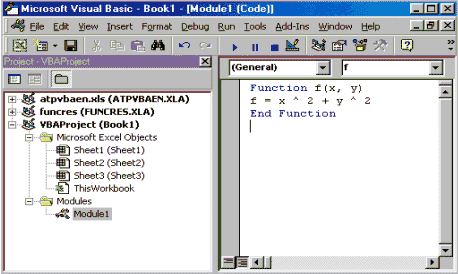Figure 7: Defining functions in Visual Basic

Alternatively, after starting Excel press <Alt> + <F11>. This will start a new program that gives access to all active Excel macros. In order to define a function one must create a module. This is done by selecting the menu item Insert and then selecting the sub-item Module. This will open an area where text may be entered. After entering the function definition the Visual Basic Editor should look something like Figure 6. After doing this we can return to the spreadsheet by closing the Visual Basic editor. Entering =f(2;2) into (note3) any cell returns the correct value 8.

### Notes

* P.O. Box 5044, N-1432 Ås, Norway, Phone: (+47) 64 94 86 24, Fax: (+47) 64 94 88 80, e-mail: eric.naevdal@isf.nlh.no. The author is grateful to an anonymous referee for finding errors and providing useful comments. Responsibility for remaining errors and omissions remain with the author. Funding for this paper came from the Norwegian Research Council, Grant #135680/703.

1. In the Solver bundled with Excel there are in built restrictions on the number of variables and constraints that the problem can have. Versions of the Solver that handles larger problems are commercially available.

2. This code could undoubtedly be written more efficiently. But not much simpler.

3. This assumes that semi-colon is the sign that Windows recognise as a list separator. If semi-colon between the arguments does not work, try with a comma.

```

```